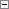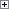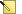Adds a text string to a BigText variable.

Parameters

BigText

Type: BigText

The BigText to which the text string will be added.

Variable

Type: BigText, Code, or Text

The string that will be added to the BigText variable. If this parameter is empty, then the BigText variable is not modified.

Position

Type: Integer

This is an optional parameter that defines the position in the BigText variable where the string is inserted. If this parameter is omitted, then the string is added at the end of the BigText variable. If this parameter is less than one, then a run-time error occurs. If this parameter is greater than the length of the BigText variable, then the string is added at the end of the BigText variable.Remarks

Variable can be inserted anywhere in BigText or added at the end of the BigText.

The first character in a BigText variable is position 1.

To delete the content in a BigText variable, use the CLEAR Function. The following code shows the syntax for the function: CLEAR(BigText)Note
If you use ADDTEXT to add multiple BigText strings to what is presented as a single string, you can experience performance problems. The same applies to other repetitive uses of ADDTEXT. This is due to the implementation of the BigText data type, which relies on a String object that is immutable. You can avoid this issue by refactoring the code to reduce the number of additions or deletions. Alternatively, you can change your implementation to use the System.Text.StringBuilder class instead. For more information, see Immutability and the StringBuilder Class in the MSDN Library.Example

The following examples show how to use the ADDTEXT function. The specified text is inserted into the BigText string at the specified position. In these examples, the initial content of the BigText variable is ABCDEFG. These examples require that you create the following variables.

Variable name DataType

MyBigText

BigText

The following example inserts the string 'ZZZ' after the character B in the MyBigText variable because 3 is specified for Position.Copy Code
// Example 1
MyBigText.ADDTEXT('ZZZ', 3); // Returns the subtext ABZZZCDEFG.Example

The following example appends the string 'ZZZ' at the end of the MyBigText variable because the number specified for Position is greater than the length of the MyBigText variable.Copy Code
// Example 2
MyBigText.ADDTEXT('ZZZ', 15); // Returns the subtext ABCDEFGZZZ.Example

In the following example, the content of the MyBigText variable is unchanged because the specified variable is an empty string.Copy Code
// Example 3ExampleCopy Code